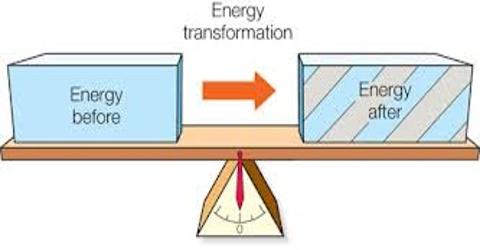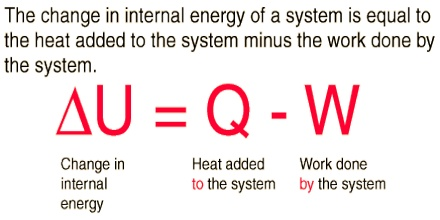# Mathematical Formulation of the First Law of Thermodynamics

Mathematical Formulation of the First Law of Thermodynamics

When heat is applied to a system, the internal energy of the system will increase if no work is done. Similarly, the energy of a system may be increased by doing work on the system in absence of heat, e.g., by rubbing two objects together, or passing electricity though a resistor. If heat and work processes occur simultaneously, the net change in internal energy of a system is equal to the algebraic sum of the energy gained or lost as heat, and energy gained or lost due to work done on or in the system, w. In other words;

∆U = q + w … … … (1)

For infinitesimally small changes equation (1) may he written as:

dU = dq + dw … … … (2)

(where, dU represents an infinitesimally small change in internal energy and similarly for q and w). It must, however, be pointed out that dq and dw do not represent differences between final and initial states.Whereas, for two given stales ∆U or dU is fixed and is always equal to dq + dw, the value of dq and dw depend on the manner in which the change from one state to another is brought about. The term dq and dw, are not regarded as perfect or exact differentials. So we should not indicate heat absorbed and work done as dq and dw, respectively and these are simply represented by q and w respectively. It is only the state properties or the state functions which are regarded as exact differentials.

Equation (2) should, therefore be written as,

dU = q + w … … … (3)

If the work done by the system is simply mechanical, i.e., work at expansion, then w = P.dV, where P is the pressure and dV is the infinitesimal increase in volume.

Equation (3) then becomes

dU = q – P.dV … … … (4)

In equation (4), the work done by the system P.dV is represented by a negative sign, because work of expansion is done by the system.